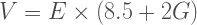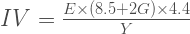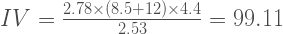# Finding intrinsic value: The Graham Formula

March 16th, 2013

There are differing views on how to find the intrinsic value of a particular stock. In The Intelligent Investor, Benjamin Graham suggested a rule of thumb method for, if not getting an exact price, at least providing a filter.

## Benjamin Graham’s original formula

The original formula for finding the value of a share involved multiplying the current earnings per share by the sum of 8.5 and twice the anticipated growth rate. That is:$V=E\times(8.5+2G)$

where  E = earnings per share and G = the anticipated growth rate over a projected period (normally 10 years).

Graham thought that as the choice for the investor was between putting money into common stocks or into the greater safety of bonds, it was appropriate to take into account the rate of interest paid on a first quality bond in determining the intrinsic value of a stock. In devising his formula, Graham took into account the then prevailing (1962) rate on triple A corporate bonds listed on the New York Stock Exchange  of 4.4 per cent.

## The Graham modified formula

As interest rates fluctuate, it became necessary for the formula to be adapted. The modified formula is:$IV=\frac{E\times(8.5+2G)\times4.4}{Y}$

where:

IV = Intrinsic value
E= Earnings per share
G= expected growth rate
Y= the current yield of triple A rated corporate bonds

## Finding the input values

The current earnings per share of the target company are available from the latest company balance sheets or from financial information providers like ADVFN.

The anticipated growth rate is difficult to determine and becomes a matter of subjective judgment. Nobody, least of all brokers and investment advisers, can predict exact future rates of growth even in the short to medium term. It is for this reason that Graham came up with the margin of safety concept: to allow for potential errors in calculating the expected growth rate.

Investors, in calculating anticipated growth rates, should take into account:

• Growth rates in EPS over the past ten years, cross checked against growth rates over the past five years
• Whether these growth rates are consistent or erratic, and whether they show an upward or at least a static trend
• Economic and industry trends
• The extent of competition for the products of the business and the likelihood of new entrants into the business markets
• Continuity and capability of company management

All these factors are part of Warren Buffett’s investment principles.

The yield rate chosen is again subjective but less so. Graham chose the figure of 4.4 because this was the triple A corporate bond rate prevailing at the time that he wrote. He completed the formula in later years to allow for the fact that the yield rates on bonds had changed.

While we have reservations about the concept of triple A rating as an investment guide (who can forget that the credit rating agencies gave triple A ratings to many of the securities that lead to the recent financial panics of 2007 and 2008), we do not argue with Graham’s use of the AAA bond rate as his yield benchmark. After all, the idea behind his formula was to determine whether an investor would be better off putting money into a particular stock or seeking the security and lesser risk of a prime bond.

As an alternative, investors using the Graham formula might prefer to substitute the yield rates for government issued securities. In the United States, an appropriate yield rate would be that for 10 year Treasury Bills or, in looking at shares listed on the stock exchanges of other countries, assuming a regular and orderly economy, the yield rate on similar bonds guaranteed by the government of that country.

Even so, this still remains very subjective. For example, the average yield on 10 year Treasury Notes from 1900 to date is about 4 per cent but in the last forty years, the average has been 6.6 per cent.

The Graham formula suggests that you should use the current rate because you are making your investment decision on today’s values, but we suppose if you really want to build in a safety margin, you could use the higher average yield of 6.6 per cent. This would, however, limit your investment options.

## Applying the margin of safety

One way of doing this is to divide the intrinsic value produced by the current price of the stock. If the result is greater than 1, the stock is selling below intrinsic value. The higher the number, the greater is the margin. If the result is less than 1, the stock is selling at a price greater than its intrinsic value.

Warren Buffett has on occasions suggested that the correct margin of safety should be about 25 per cent and this seems a good figure to us but again it would depend upon how much confidence we had in the assumed growth rates. If we were highly confident, we might accept a smaller margin and vice versa. If less confident, then the discount figure used should be greater.

## How the Graham formula works

Using a hypothetical example, suppose that the current earnings per share of Company XYZ is $2.78 and that, taking into account all the factors that you should take into account, you conservatively calculate the projected growth rate at 6 per cent. Suppose also that the current selling price of the stock is$70.55. At the time of writing this post (March 2013) the yield on 10 year American Triple A corporate 10 year bonds is 2.53 per cent.

The values then are E = 2.78, G = 6 (therefore 2G = 12) and Y = 2.53. So:$IV=\frac{2.78\times(8.5+12)\times4.4}{2.53}=99.11$

Using the Graham modified formula, the intrinsic value of a share in XYZ Company then is $99.11. ## Incorporating the margin of safety To incorporate a margin of safety, we can now divide the intrinsic value (99.11) by the current price of the stock (70.55). We get 1.40 which is a positive ratio and indicates a buy. If we adopt Buffett’s stated margin of safety value of 25 per cent, we would get a buy price of$74.33(99.11 x .75), again a buy.

Even allowing for the current historically low interest rates, the Graham formula suggests that this hypothetical stock is selling at below intrinsic value.

## Using the Graham formula

We don’t suggest that this formula is the best, the most correct or indeed the only way of working out the intrinsic value of a share. It was offered by Graham as a rule of thumb method fifty years ago. Its most appropriate use is, we would think, as a filtering and checking mechanism.